You are currently offline. Some features of the site may not work correctly.

# Mathematics of cyclic redundancy checks

Known as: Mathematics of CRCs, Mathematics of crc, Mathmatics of CRCs
The cyclic redundancy check (CRC) is based on division in the ring of polynomials over the finite field GF(2) (the integers modulo 2), that is, the… Expand
Wikipedia

## Papers overview

Semantic Scholar uses AI to extract papers important to this topic.
2012
2012
We derive sufficient conditions under which all but two zeros of reciprocal polynomials lie on the unit circle, and specify the… Expand
•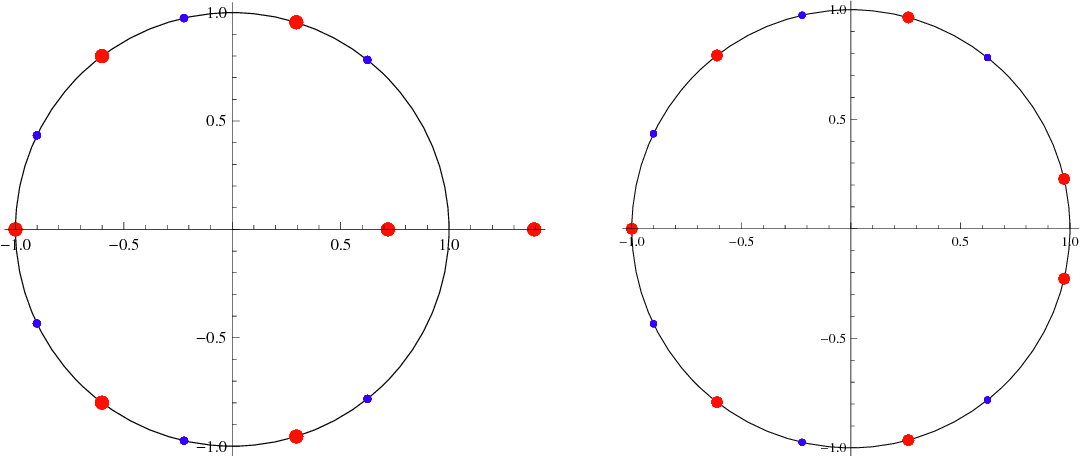•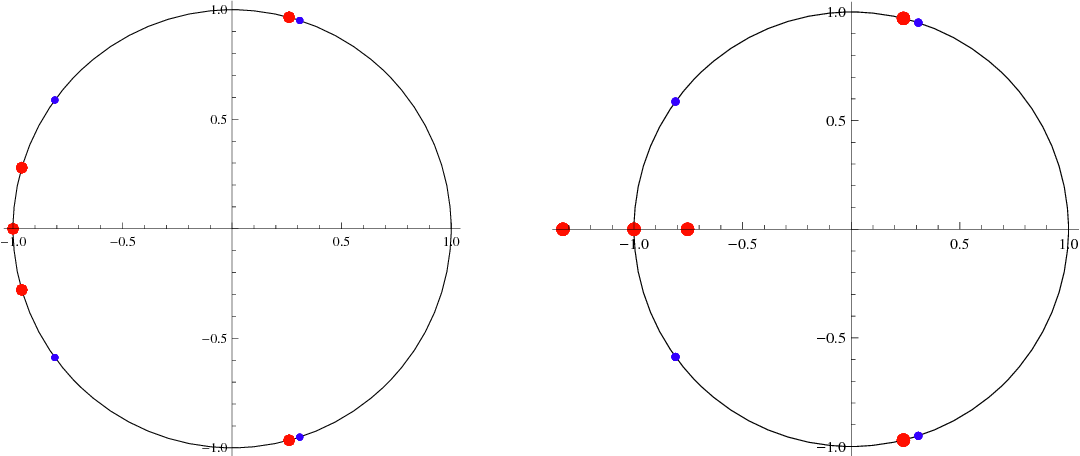Is this relevant?
2010
2010
The measure of a monic polynomial is the product of the absolute value of the roots which lie outside and on the unit circle. We… Expand
•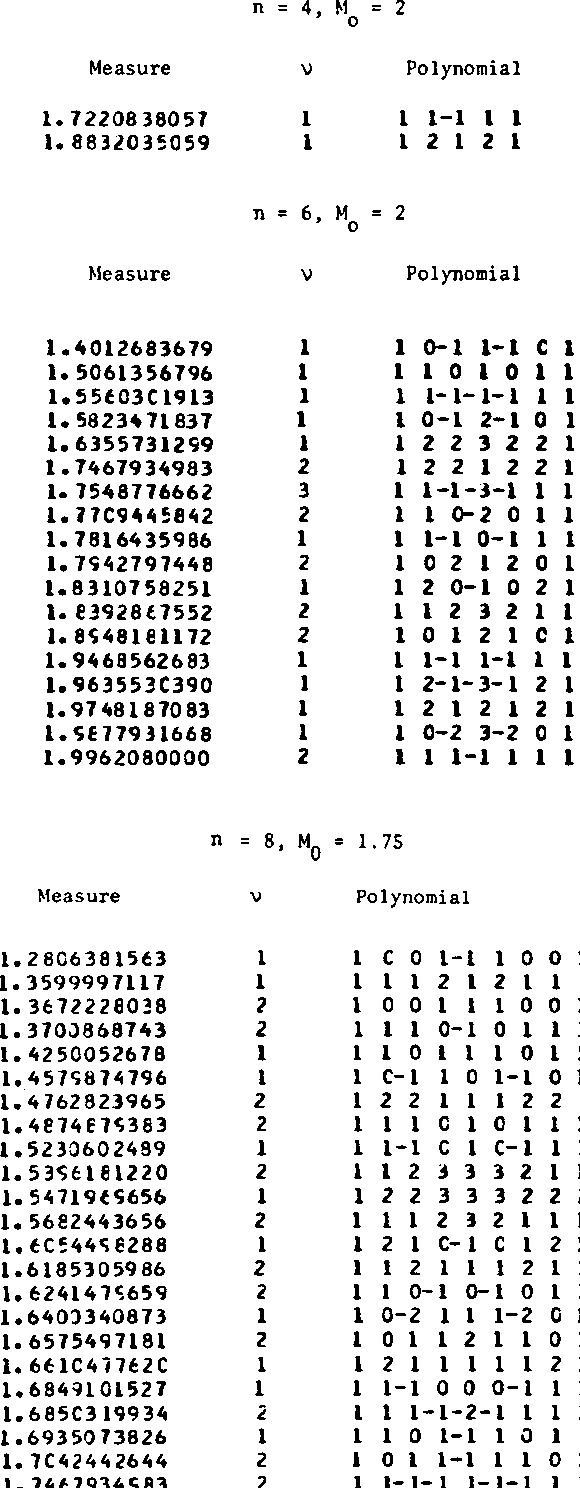•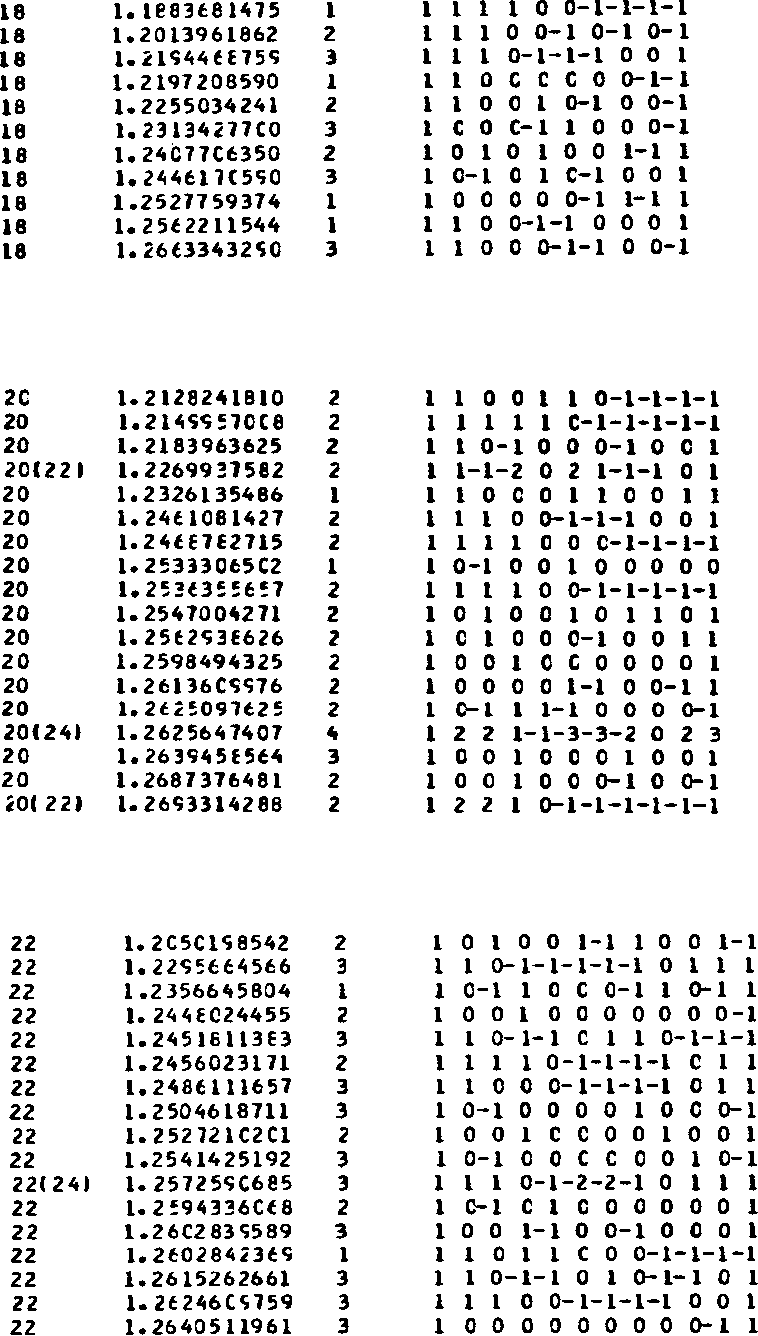Is this relevant?
2007
2007
For integral self-reciprocal polynomials P(z) and Q(z) with all zeros lying on the unit circle, does there exist integral self… Expand
Is this relevant?
2005
2005
• Helmut Meyn
• Applicable Algebra in Engineering, Communication…
• 2005
• Corpus ID: 7965318
AbstractThe transformationf(x)↦fQx≔deg(f)f(x + 1/x) for f(x)∈ \$\$\mathbb{F}_q [x]\$\$ is studied. Simple criteria are given for the… Expand
Is this relevant?
2003
2003
• 2003
• Corpus ID: 14335777
The first author  proved that all zeros of the reciprocal polynomial Pm(z) = m ∑ k=0 Akz k (z ∈ C), of degreem ≥ 2 with real… Expand
•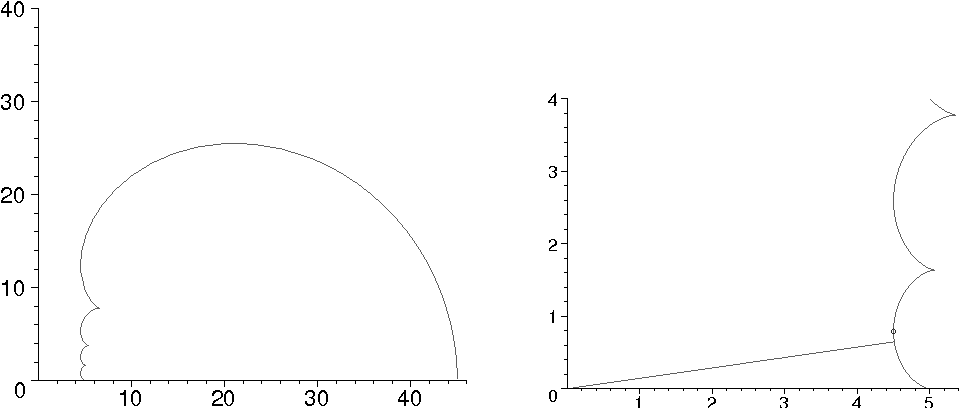Is this relevant?
2002
2002
The purpose of this paper is to show that all zeros of the reciprocal polynomial Pm(z) = m ∑ k=0 Akz k (z ∈ C) of degree m ≥ 2… Expand
Is this relevant?
1996
1996
• Autom.
• 1996
• Corpus ID: 39238396
Abstract A ‘switched’ or ‘multimode’ system is one that can switch between various modes of operation. We consider here switched… Expand
•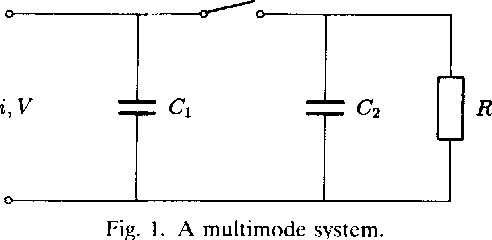Is this relevant?
1991
1991
We investigate a nonstandard output convention for sorting. Specifically, the input elements are to be returned not in an array… Expand
Is this relevant?
1989
1989
• 1989
• Corpus ID: 13189567
The reciprocal f (x) of a polynomial f(x) of degree n is defined by f (x) = xf(1/x). A polynomial is called self-reciprocal if it… Expand
Is this relevant?
1980
1980
The measure of a monic polynomial is the product of the absolute value of the roots which lie outside and on the unit circle. We… Expand
•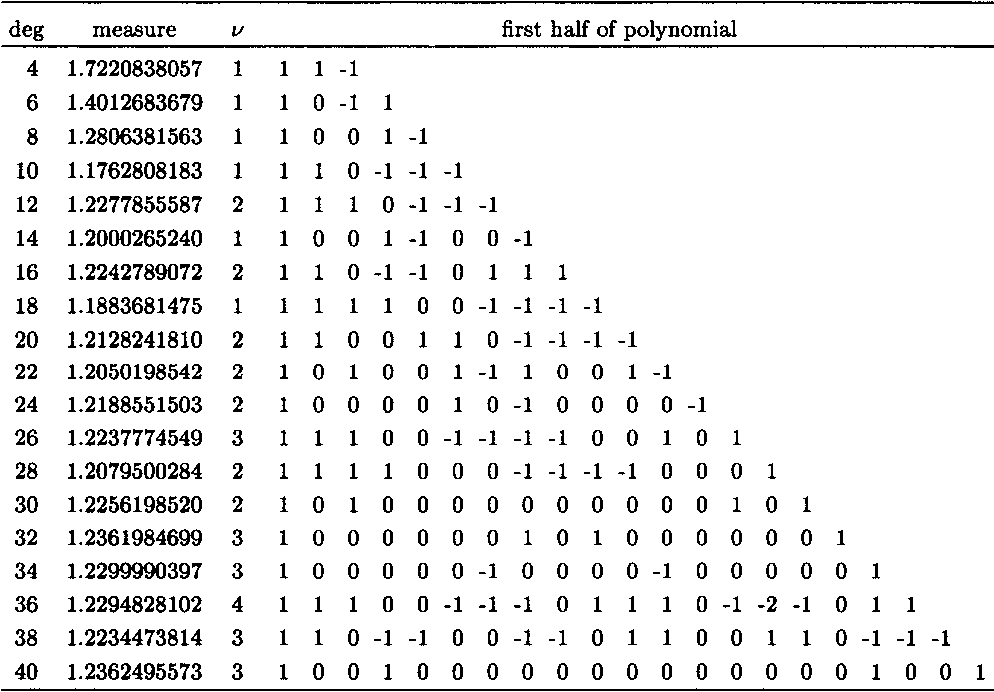Is this relevant?